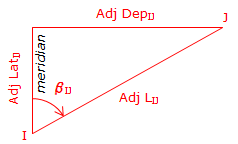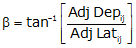Article Index

Regardless of the adjustment method applied, changing a line's Lat and Dep will in turn change the length and direction of the line.Figure E-4 Adjusted Length and Direction

The adjusted length can be computed from the Pythagorean theorem:Equation E-5

Computing direction is a two-step process: (1) Determine β, the angle from the meridian to the line (2) Convert β into a direction based on the line's quadrant.

To determine β:Equation E-6

β falls in the range of -90° to +90°.

The sign on β indicates the direction of turning from the meridian: (+) is clockwise, (-) is counter-clockwise. The combined signs on the adjusted Lat and Dep will identify the line's quadrant.

Figure E-5 shows the quadrant and direction computation for the various mathematic combinations of the adjusted Lat and Dep:Figure E-5 Converting ß to a Direction